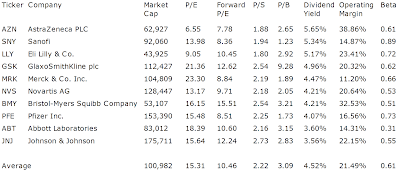## Pages

### The 10 Best Dividend Paying Drug Stocks

Best Dividend Paying Drug Stocks by Dividend Yield - Stock, Capital, Investment. Here is a current sheet stocks from major drug manufacturing industry with positive dividend yield. 36 stocks are listed within the industry and they have a total market capitalization of USD 39.4 trillion. Only 10 of them pay dividends.

Here are the 3 top stocks by dividend yield:
(Subscribe my Blog via RSS Feed or E-Mail. Alternative, you can follow me on Facebook or Twitter)

AstraZeneca (NYSE:AZN) has a market capitalization of \$63.08 billion. The company employs 61,100 people, generates revenues of \$33,269.00 million and has a net income of \$8,081.00 million. The firm’s earnings before interest, taxes, depreciation and amortization (EBITDA) amounts to \$13,380.00 million. Because of these figures, the EBITDA margin is 40.22 percent (operating margin 34.55 percent and the net profit margin finally 24.29 percent).

The total debt representing 16.43 percent of the company’s assets and the total debt in relation to the equity amounts to 39.73 percent. Due to the financial situation, the return on equity amounts to 36.71 percent. Finally, earnings per share amounted to \$7.29 of which \$2.55 were paid in form of dividends to shareholders.

Here are the price ratios of the company: The P/E ratio is 6.56, Price/Sales 1.87 and Price/Book ratio 2.87. Dividend Yield: 5.71 percent. The beta ratio is 0.61.Long-Term Stock Chart Of AstraZeneca plc (ADR) (Click to enlarge)

Sanofi (NYSE:SNY) has a market capitalization of \$91.59 billion. The company employs 101,575 people, generates revenues of \$44,035.57 million and has a net income of \$5,989.17 million. The firm’s earnings before interest, taxes, depreciation and amortization (EBITDA) amounts to \$14,726.18 million. Because of these figures, the EBITDA margin is 33.44 percent (operating margin 18.59 percent and the net profit margin finally 13.60 percent).

The total debt representing 9.69 percent of the company’s assets and the total debt in relation to the equity amounts to 15.56 percent. Due to the financial situation, the return on equity amounts to 10.78 percent. Finally, earnings per share amounted to \$2.44 of which \$1.72 were paid in form of dividends to shareholders.

Here are the price ratios of the company: The P/E ratio is 13.96, Price/Sales 2.09 and Price/Book ratio 1.22. Dividend Yield: 5.16 percent. The beta ratio is 0.89.Long-Term Stock Chart Of Sanofi SA (ADR) (Click to enlarge)

Eli Lilly & (NYSE:LLY) has a market capitalization of \$43.93 billion. The company employs 38,380 people, generates revenues of \$23,076.00 million and has a net income of \$5,069.50 million. The firm’s earnings before interest, taxes, depreciation and amortization (EBITDA) amounts to \$7,793.60 million. Because of these figures, the EBITDA margin is 33.77 percent (operating margin 28.28 percent and the net profit margin finally 21.97 percent).

The total debt representing 22.34 percent of the company’s assets and the total debt in relation to the equity amounts to 55.77 percent. Due to the financial situation, the return on equity amounts to 46.20 percent. Finally, earnings per share amounted to \$4.19 of which \$1.96 were paid in form of dividends to shareholders.

Here are the price ratios of the company: The P/E ratio is 9.06, Price/Sales 1.88 and Price/Book ratio 3.48. Dividend Yield: 5.23 percent. The beta ratio is 0.72.Long-Term Stock Chart Of Eli Lilly & Co. (Click to enlarge)

Take a closer look at the full table of best dividend paying drug stocks. The average price to earnings ratio (P/E ratio) amounts to 15.3 while the forward P/E ratio is 10.5. The dividend yield has a value of 4.5 percent. Price to book ratio is 3.1 and price to sales ratio 2.2. The operating margin amounts to 21.5 percent.

Here is the table with some fundamentals (TTM):The 10 Best Dividend Paying Drug Stocks (Click to enlarge)

Related stock ticker symbols:
AZN, SNY, LLY, GSK, MRK, NVS, BMY, PFE, ABT, JNJ

Selected Articles: